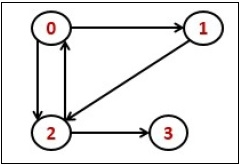# Transitive closure of a Graph

Data StructureGraph AlgorithmsAlgorithms

#### Big Data Hadoop

Best Seller

89 Lectures 11.5 hours

#### Practical Data Science using Python

22 Lectures 6 hours

#### Data Science and Data Analysis with Python

50 Lectures 3.5 hours

Transitive Closure it the reachability matrix to reach from vertex u to vertex v of a graph. One graph is given, we have to find a vertex v which is reachable from another vertex u, for all vertex pairs (u, v).The final matrix is the Boolean type. When there is a value 1 for vertex u to vertex v, it means that there is at least one path from u to v.

## Input and Output

Input:
1 1 0 1
0 1 1 0
0 0 1 1
0 0 0 1

Output:
The matrix of transitive closure
1 1 1 1
0 1 1 1
0 0 1 1
0 0 0 1

## Algorithm

transColsure(graph)

Input: The given graph.
Output: Transitive Closure matrix.

Begin
copy the adjacency matrix into another matrix named transMat
for any vertex k in the graph, do
for each vertex i in the graph, do
for each vertex j in the graph, do
transMat[i, j] := transMat[i, j] OR (transMat[i, k]) AND transMat[k, j])
done
done
done
Display the transMat
End



<2>Example

#include<iostream>
#include<vector>
#define NODE 4
using namespace std;

/* int graph[NODE][NODE] = {
{0, 1, 1, 0},
{0, 0, 1, 0},
{1, 0, 0, 1},
{0, 0, 0, 0}
}; */

int graph[NODE][NODE] = {
{1, 1, 0, 1},
{0, 1, 1, 0},
{0, 0, 1, 1},
{0, 0, 0, 1}
};

int result[NODE][NODE];

void transClosure() {
for(int i = 0; i<NODE; i++)
for(int j = 0; j<NODE; j++)
result[i][j] = graph[i][j];    //initially copy the graph to the result matrix
for(int k = 0; k<NODE; k++)
for(int i = 0; i<NODE; i++)
for(int j = 0; j<NODE; j++)
result[i][j] = result[i][j] || (result[i][k] && result[k][j]);
for(int i = 0; i<NODE; i++) {          //print the result matrix
for(int j = 0; j<NODE; j++)
cout << result[i][j] << " ";
cout << endl;
}
}

int main() {
transClosure();
}

## Output

1 1 1 1
0 1 1 1
0 0 1 1
0 0 0 1